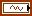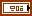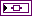# TSA Time-Cepstrum VI

LabVIEW 2014 Advanced Signal Processing Toolkit Help

Edition Date: June 2014

Part Number: 372656C-01

»View Product InfoDownload Help (Windows Only)

Owning Palette: Correlation and Spectral Analysis VIs

Computes the single-sided time-cepstrum of a univariate time series by using a sliding window. You can use the resulting time-cepstrum to detect time-varying periodic components of a time series. Wire data to the Xt input to determine the polymorphic instance to use or manually select the instance.

Example

Use the pull-down menu to select an instance of this VI.

 Select an instance TSA Time-Cepstrum (Waveform)TSA Time-Cepstrum (Array)

## TSA Time-Cepstrum (Waveform)method specifies whether this VI uses the FFT-based or the autoregressive (AR) model-based method to compute the real cepstrum. The default is FFT.quefrency offset specifies the offset, in seconds, of the quefrency. This VI returns the real cepstrum at a certain time whose quefrency is larger than the offset value. The default is 0.Xt specifies the univariate time series.time-quefrency sampling info specifies information about the density and the size of the time-cepstrum.time steps specifies the sampling period, in number of samples, along the time axis in the joint time-quefrency domain. The default is –1, which specifies that this VI adjusts time steps automatically so that no more than 512 rows exist in the cepstrogram. National Instruments recommends that you set time steps such that the number of rows in cepstrogram does not exceed 512. If you specify a small value for time steps, this VI might return a large cepstrogram, which requires a long computation time and more memory. If you need a small sampling period to observe more details and the signal length is long, divide the signal into smaller segments and compute the cepstrogram for each segment. If the signal is oversampled, you also can downsample the signal. The scale info output contains the actual sampling period, in seconds, along the time axis of the cepstrogram.quefrency bins specifies the number of bins along the quefrency axis of the cepstrogram to sample the time series in the joint time-quefrency domain. quefrency bins must be greater than 0. The scale info output contains the actual sampling period, in seconds, along the quefrency axis of the cepstrogram. The default is 512.window info specifies the information about the sliding window that divides the time series Xt into subsequences.type specifies the time-domain window that this VI applies to the time series.

 0 None 1 Hanning (default) 2 Hamming 3 Blackman-Harris 4 Exact Blackman 5 Blackman 6 Flat Top 7 4-Term B-Harris 8 7-Term B-Harris 9 Low Sidelobe 10 Gaussianlength specifies the length of the window in samples. The default is –1, which indicates that the window length equals the length of the input time series.error in describes error conditions that occur before this node runs. This input provides standard error in functionality.AR setting specifies the settings for the autoregressive (AR) model. This option is valid only when the method is AR Model.AR method specifies the method this VI uses to estimate the autoregressive (AR) model.

 0 Forward-Backward (default)—Computes the AR coefficients by minimizing the least-square errors of the forward and backward predictions. 1 Least-Squares—Computes the AR coefficients by minimizing the least-square errors of the forward predictions. 2 Yule-Walker—Computes the AR coefficients by solving the Yule-Walker functions based on the forward predictions. 3 Burg-Lattice—Computes the AR coefficients using the Levinson-Durbin recursion based on the forward and backward predictions. The Levinson-Durbin recursion uses the arithmetic average. 4 Geometric-Lattice—Computes the AR coefficients using the Levinson-Durbin recursion based on the forward and backward predictions. The Levinson-Durbin recursion uses the geometric average.AR order specifies the order of the autoregressive (AR) model. The value of AR order must be greater than 0. The default is 4.cepstrogram returns the time-quefrency representation of the time series Xt. Each row corresponds to the real cepstrum at a certain time. The number of rows in cepstrogram equals the signal length divided by time steps. You can use the TSA Get Time and Quefrency Scale Info VI to compute the time scale information and the quefrency scale information of the time-quefrency representation.scale info returns the time scale and quefrency scale information of the time-quefrency representation, including the time offset, the time interval between every two contiguous rows, the quefrency offset, and the quefrency interval between every two contiguous columns of the cepstrogram.overlap returns the overlap, in percentage, of the sliding window this VI applies to the time series.error out contains error information. This output provides standard error out functionality.

## TSA Time-Cepstrum (Array)method specifies whether this VI uses the FFT-based or the autoregressive (AR) model-based method to compute the real cepstrum. The default is FFT.quefrency offset specifies the offset, in seconds, of the quefrency. This VI returns the real cepstrum at a certain time whose quefrency is larger than the offset value. The default is 0.Xt specifies the univariate time series.time-quefrency sampling info specifies information about the density and the size of the time-cepstrum.time steps specifies the sampling period, in number of samples, along the time axis in the joint time-quefrency domain. The default is –1, which specifies that this VI adjusts time steps automatically so that no more than 512 rows exist in the cepstrogram. National Instruments recommends that you set time steps such that the number of rows in cepstrogram does not exceed 512. If you specify a small value for time steps, this VI might return a large cepstrogram, which requires a long computation time and more memory. If you need a small sampling period to observe more details and the signal length is long, divide the signal into smaller segments and compute the cepstrogram for each segment. If the signal is oversampled, you also can downsample the signal. The scale info output contains the actual sampling period, in seconds, along the time axis of the cepstrogram.quefrency bins specifies the number of bins along the quefrency axis of the cepstrogram to sample the time series in the joint time-quefrency domain. quefrency bins must be greater than 0. The scale info output contains the actual sampling period, in seconds, along the quefrency axis of the cepstrogram. The default is 512.window info specifies the information about the sliding window that divides the time series Xt into subsequences.type specifies the time-domain window that this VI applies to the time series.

 0 None 1 Hanning (default) 2 Hamming 3 Blackman-Harris 4 Exact Blackman 5 Blackman 6 Flat Top 7 4-Term B-Harris 8 7-Term B-Harris 9 Low Sidelobe 10 Gaussianlength specifies the length of the window in samples. The default is –1, which indicates that the window length equals the length of the input time series.error in describes error conditions that occur before this node runs. This input provides standard error in functionality.AR setting specifies the settings for the autoregressive (AR) model. This option is valid only when the method is AR Model.AR method specifies the method this VI uses to estimate the autoregressive (AR) model.

 0 Forward-Backward (default)—Computes the AR coefficients by minimizing the least-square errors of the forward and backward predictions. 1 Least-Squares—Computes the AR coefficients by minimizing the least-square errors of the forward predictions. 2 Yule-Walker—Computes the AR coefficients by solving the Yule-Walker functions based on the forward predictions. 3 Burg-Lattice—Computes the AR coefficients using the Levinson-Durbin recursion based on the forward and backward predictions. The Levinson-Durbin recursion uses the arithmetic average. 4 Geometric-Lattice—Computes the AR coefficients using the Levinson-Durbin recursion based on the forward and backward predictions. The Levinson-Durbin recursion uses the geometric average.AR order specifies the order of the autoregressive (AR) model. The value of AR order must be greater than 0. The default is 4.sampling rate specifies the sampling rate, in hertz, of the univariate time series Xt. The default is 1.cepstrogram returns the time-quefrency representation of the time series Xt. Each row corresponds to the real cepstrum at a certain time. The number of rows in cepstrogram equals the signal length divided by time steps. You can use the TSA Get Time and Quefrency Scale Info VI to compute the time scale information and the quefrency scale information of the time-quefrency representation.scale info returns the time scale and quefrency scale information of the time-quefrency representation, including the time offset, the time interval between every two contiguous rows, the quefrency offset, and the quefrency interval between every two contiguous columns of the cepstrogram.overlap returns the overlap, in percentage, of the sliding window this VI applies to the time series.error out contains error information. This output provides standard error out functionality.

## Example

Refer to the Bearing Time-Cepstrum Analysis VI in the labview\examples\Time Series Analysis\TSAApplications directory for an example of using the TSA Time-Cepstrum VI.